# Fitting Hyperbolic Cosine Model (HCM) For Unfolding Dichotomous Responses using Stan

In this post, I do a quick exercise on fitting the hyperbolic cosine model using Stan. The information about this model can be found in Andrich & Luo (1993). The most interesting part is an “Aha!” moment when I discover the bimodality of posterior distribution due to lack of directional constrain.

Cengiz Zopluoglu (University of Miami)
12-15-2019

In this post, I do a quick exercise on fitting the hyperbolic cosine model using Stan. The information about this model can be found in Andrich & Luo (1993). The paper by Johnson and Junker (2003) also provide a detailed treatment of estimating this particular model.

The hyperbolic cosine model (HCM) is described as

$P(X_{ij}=1)=\frac{exp(\gamma_j)}{exp(\gamma_j)+2cosh(\theta_i-\beta_j)}.$ In this model,

• $$\theta_i$$ is the latent variable for the person $$i$$,
• $$\beta_j$$ is the location of item $$j$$ on the latent continuum, and
• $$\gamma_j$$ is the unit parameter for the item $$j$$.

While $$\gamma_j$$ is an item specific parameter in the equation above, it can be fixed to the same value across items (Simplified HCM). The model was primarily developed to measure attitudes, and $$P(X_{ij}=1)$$ is used as the probability of endorsing an item (endorsing=1, not endorsing = 0).

In order to understand the model, let’s check how the probability of endorsement changes as a function of the latent variable and how this is different than dichotomous IRT models for correct/incorrect scoring. Below are the item characteristic curve for three hypothetical items with $$\beta= -1$$ and different unit parameter ($$\gamma={1,2,3}$$).


gamma <- c(1,2,3)
beta  <- -1
theta <- seq(-4,4,.01)

p1 <- exp(gamma)/(exp(gamma)+2*cosh(theta-beta))
p2 <- exp(gamma)/(exp(gamma)+2*cosh(theta-beta))
p3 <- exp(gamma)/(exp(gamma)+2*cosh(theta-beta))

plot(theta,p1,xlab="Theta",ylab="Probability of Agreement",type="l",ylim=c(0,1))
points(theta,p2,xlab="Theta",ylab="Probability of Agreement",type="l",lty=2)
points(theta,p3,xlab="Theta",ylab="Probability of Agreement",type="l",lty=3)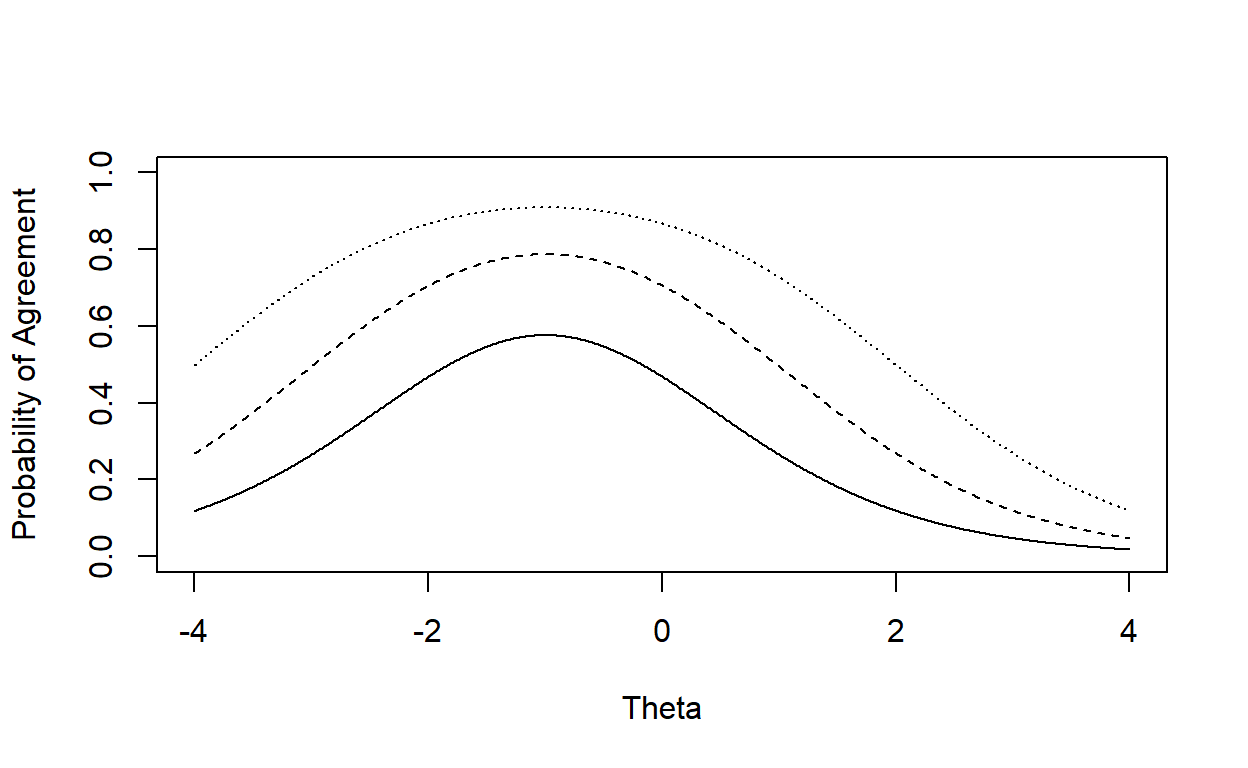The most important distinction of HCM is that the probability of endorsement does not monotonically increase as the latent variable increases. The probability reaches to its maximum when $$\theta$$ is equal to $$\beta$$, and the maximum probability of endorsement is as a function of the unit parameter, $P_{max}(X_{ij}=1)=\frac{1}{1 +2exp(-\gamma_j)}$. The more the difference between person location ($$\theta$$) and item location ($$\beta$$) is, the lower $$P(X_{ij}=1)$$ is. Also, note that $$\gamma$$ behaves like a discrimination parameter. The higher the value of the $$\gamma$$ parameter is, the more flat the item response function becomes. Higher values of $$\gamma$$ indicate less discrimination along the scale.

## Simulated Dataset

### Data Generation

First, I simulate a dataset based on HCM. I assume

• the sample size is 500,
• the number of items is 8,
• $$\beta$$ parameters are {-2,-1.5,-1,-.5,.5,1,1.5,2},
• $$\gamma$$ is equal to 0.7 for all items, and
• $$\theta$$ parameters are drawn from the standard normal distribution.

require(purrr)

set.seed(123456)

N = 500
k = 8

beta  <- c(-2,-1.5,-1,-.5,.5,1,1.5,2)

gamma <- rep(0.7,k)

theta <- rnorm(N,0,1)

d <- matrix(NA,nrow=N,ncol=k)

for(i in 1:nrow(d)){
for(j in 1:ncol(d)){

prob   = exp(gamma[j])/(exp(gamma[j])+2*cosh(theta[i] - beta[j]))
d[i,j] = (rbernoulli(1, p = prob))*1

}
}

head(d)

[,1] [,2] [,3] [,4] [,5] [,6] [,7] [,8]
[1,]    0    1    1    1    0    1    1    0
[2,]    0    1    1    0    1    0    0    0
[3,]    0    0    1    0    1    0    0    0
[4,]    1    0    1    0    1    0    1    0
[5,]    0    0    0    0    1    0    0    1
[6,]    0    1    0    0    1    1    1    1

### Fitting HCM using Stan

When I first tried to fit the model, I started with the following model syntax. Following the conventional approach in other dichotomous and polytomous IRT models, I set the mean and variance of the latent scale to 0 and 1, respectively. I tried to estimate a separate $$\beta$$ and $$\gamma$$ for each item without any additional constraints.


hcm <-'

data{
int I;                   //  number of individuals
int J;                   //  number of items
int <lower=0> Y[I,J];    //  data matrix
}

parameters {
vector[J] beta;
real mu_beta;
real<lower=0> sigma_beta;

vector[J] gamma;
real mu_gamma;
real<lower=0> sigma_gamma;

vector[I] theta;
}

model{

beta    ~ normal(mu_beta,sigma_beta);
mu_beta ~ normal(0,5);
sigma_beta ~ cauchy(0,2.5);

gamma   ~ normal(mu_gamma,sigma_gamma);
mu_gamma ~ normal(0,5);
sigma_gamma ~ cauchy(0,2.5);

theta   ~ normal(0,1);

for(i in 1:I) {
for(j in 1:J) {

real p  = exp(gamma[j])/(exp(gamma[j])+2*cosh(theta[i] - beta[j]));

Y[i,j] ~ bernoulli(p);

}}
}
'

I fitted the model using four chains with the following settings.


require(rstan)

data <- list(Y=d,
I=nrow(d),
J=ncol(d))

fit_hcm <- stan(model_code = hcm,
data       = data,
iter       = 2000,
chains     = 4,
control    = list(max_treedepth = 15,adapt_delta=0.9))

Let’s check the solution for item parameters.


print(fit_hcm,
pars = c("beta","mu_beta","sigma_beta",
"gamma","mu_gamma","sigma_gamma"),
probs = c(0.025,0.975),
digits = 3)

Inference for Stan model: 5ba394328e7553d4461ee58a0ae9eb24.
4 chains, each with iter=2000; warmup=1000; thin=1;
post-warmup draws per chain=1000, total post-warmup draws=4000.

mean se_mean    sd   2.5% 97.5% n_eff  Rhat
beta     -1.114   1.366 1.953 -2.815 2.594     2 6.579
beta     -0.788   0.970 1.401 -2.146 1.932     2 4.720
beta     -0.661   0.791 1.158 -2.013 1.616     2 3.746
beta     -0.091   0.121 0.360 -0.775 0.635     9 1.152
beta      0.281   0.365 0.587 -0.981 1.128     3 2.039
beta      0.390   0.504 0.759 -1.180 1.336     2 2.824
beta      0.789   0.962 1.391 -1.938 2.203     2 4.535
beta      1.013   1.238 1.779 -2.359 2.676     2 5.516
mu_beta     -0.040   0.011 0.661 -1.360 1.244  3729 1.001
sigma_beta   1.797   0.012 0.571  1.039 3.275  2175 0.999
gamma     0.731   0.006 0.242  0.242 1.261  1537 1.001
gamma     0.756   0.007 0.217  0.383 1.184  1005 1.002
gamma     0.968   0.009 0.237  0.656 1.516   663 1.003
gamma     0.625   0.002 0.100  0.423 0.817  2421 1.001
gamma     0.749   0.003 0.119  0.545 1.014  1998 1.003
gamma     0.756   0.004 0.137  0.526 1.052  1246 1.001
gamma     0.770   0.006 0.215  0.404 1.264  1328 1.002
gamma     0.748   0.009 0.264  0.284 1.316   962 1.004
mu_gamma     0.760   0.004 0.134  0.526 1.048  1033 1.002
sigma_gamma  0.225   0.007 0.170  0.034 0.679   558 1.005

Samples were drawn using NUTS(diag_e) at Fri Nov 29 17:58:33 2019.
For each parameter, n_eff is a crude measure of effective sample size,
and Rhat is the potential scale reduction factor on split chains (at
convergence, Rhat=1).

This was very strange. The parameter estimates were absolutely bizarre, particularly the $$\beta$$ parameters. Let’s check the posterior distribution for the item parameters.


plot(fit_hcm,
plotfun = "hist",
pars = c("beta","beta","beta","beta",
"beta","beta","beta","beta"),
inc_warmup = FALSE)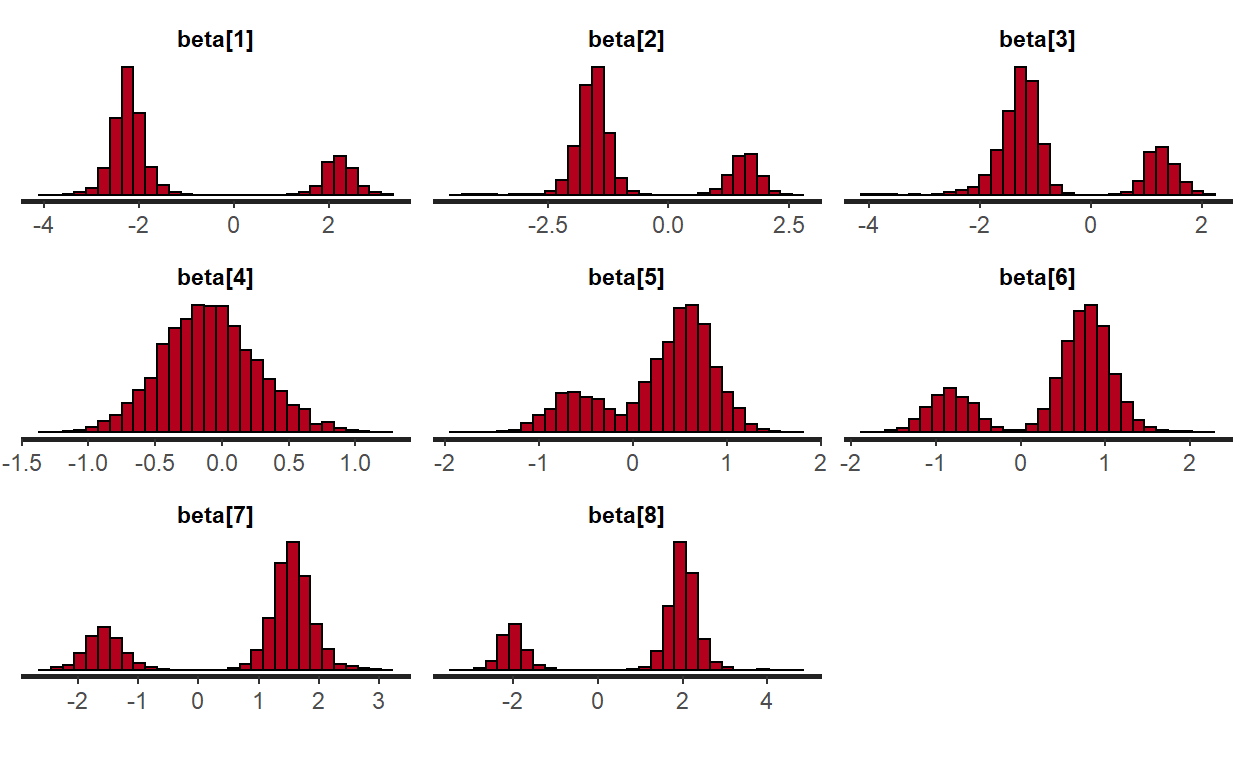plot(fit_hcm,
plotfun = "trace",
pars = c("beta"),
inc_warmup = FALSE)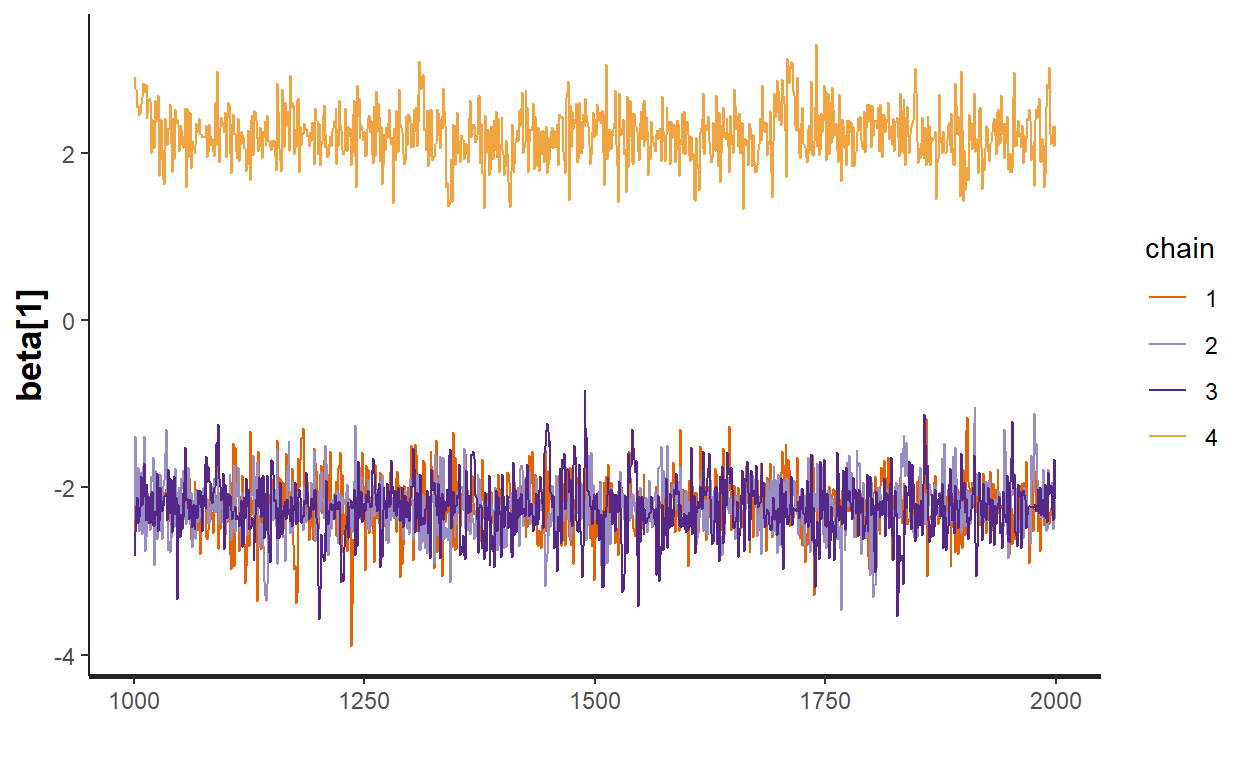This clearly signaled a problem. It took me a whole day to figure out the issue, until I read the paper by Johnson and Junker (2003, page 209-211). Without any constraint on the sign of the beta parameters, the direction of the scale is arbitrary. Chains hang out on opposite side of the continuum, leading a bimodal posterior. One needs to add a directional constrain for the beta parameters. Johnson & Junker (2003) says “Constraining the sign of a single item’s location is one method for ensuring identifiability (e.g., β1 < 0).”

I tried to do constrain the sign for one of the $$\beta$$ parameters but I realized this was also not enough. It sometimes works but most of the time the $$\beta$$ parameters with no constraint still suffer from the same issue. So, I think one has to put a constrain on each $$\beta$$ parameter. That means you have to guess the sign of the $$\beta$$ parameters before fitting the model. It is easy in this case since this is the simulated data and I know the sign of the $$\beta$$ parameter. However, in real data, this is an issue to deal with. I’ve come to a solution by first fitting the model with only one chain to have an idea about the sign of the $$\beta$$ parameters (there might be other and better ways of doing this). Once I have an idea about the sign of the parameters, then you modify the model syntax accordingly to fit the model with more chains. Let’s refit the model with only one chain and fewer iterations as all I want to see is the sign of the $$\beta$$ parameter.


fit_hcm_onechain <- stan(model_code = hcm,
data       = data,
iter       = 200,
chains     = 1,
control    = list(max_treedepth = 15,adapt_delta=0.9))

print(fit_hcm_onechain,
pars = c("beta","mu_beta","sigma_beta"),
probs = c(0.025,0.975),
digits = 3)

Inference for Stan model: 5ba394328e7553d4461ee58a0ae9eb24.
1 chains, each with iter=200; warmup=100; thin=1;
post-warmup draws per chain=100, total post-warmup draws=100.

mean se_mean    sd   2.5%  97.5% n_eff  Rhat
beta     2.238   0.029 0.320  1.608  2.767   125 1.005
beta     1.649   0.038 0.315  0.965  2.184    70 1.006
beta     1.257   0.055 0.341  0.669  1.979    39 1.021
beta     0.251   0.046 0.328 -0.383  0.819    51 1.004
beta    -0.553   0.027 0.278 -1.138 -0.062   110 0.990
beta    -0.815   0.038 0.291 -1.295 -0.163    58 0.992
beta    -1.610   0.042 0.427 -2.485 -0.874   103 0.992
beta    -1.987   0.031 0.301 -2.508 -1.327    97 1.002
mu_beta    -0.034   0.102 0.842 -2.122  1.296    68 0.990
sigma_beta  1.863   0.077 0.540  1.191  3.069    49 0.990

Samples were drawn using NUTS(diag_e) at Fri Nov 29 18:01:15 2019.
For each parameter, n_eff is a crude measure of effective sample size,
and Rhat is the potential scale reduction factor on split chains (at
convergence, Rhat=1).

So, this solution suggests that the $$\beta$$ parameters from Item 1 to Item 4 are positive and the parameters from Item 5 to Item 8 are negative. Note that this is exact opposite of the true signs I used when generating data. Again, the direction of the scale is totally arbitrary. So, all I need to know is that the Item 1 - Item 4 should have the same sign while Item 5 - Item 8 should have the opposite sign. It is up to me which sign I assign to which set of items. After having this information, I modified the syntax below accordingly and constrained the sign of each $$\beta$$ parameter.


hcm2 <-'

data{
int I;                   //  number of individuals
int J;                   //  number of items
int <lower=0> Y[I,J];    //  data matrix
}
parameters {
vector<upper=0> beta1;
vector<lower=0> beta2;
real mu_beta;
real<lower=0> sigma_beta;

vector[J] gamma;
real mu_gamma;
real<lower=0> sigma_gamma;

vector[I] theta;
}

model{

mu_gamma ~ normal(0,5);
sigma_gamma ~ cauchy(0,2.5);
gamma   ~ normal(mu_gamma,sigma_gamma);

mu_beta ~ normal(0,5);
sigma_beta ~ cauchy(0,2.5);
beta1    ~ normal(mu_beta,sigma_beta);
beta2    ~ normal(mu_beta,sigma_beta);

theta    ~ normal(0,1);

for(i in 1:I) {
for(j in 1:4) {
real p1  = exp(gamma[j])/(exp(gamma[j])+2*cosh(theta[i] - beta1[j]));
Y[i,j] ~ bernoulli(p1);
}}

for(i in 1:I) {
for(j in 5:8) {
real p2  = exp(gamma[j])/(exp(gamma[j])+2*cosh(theta[i] - beta2[j-4]));
Y[i,j] ~ bernoulli(p2);
}}

}
'

fit_hcm2 <- stan(model_code = hcm2,
data       = data,
iter       = 2000,
chains     = 4,
control    = list(max_treedepth = 15,adapt_delta=0.9))

print(fit_hcm2,
pars = c("beta1","beta2","mu_beta","sigma_beta","gamma"),
probs = c(0.025,0.975),
digits = 3)

Inference for Stan model: 47cab98047122166c612533d29d2f0ce.
4 chains, each with iter=2000; warmup=1000; thin=1;
post-warmup draws per chain=1000, total post-warmup draws=4000.

mean se_mean    sd   2.5%  97.5% n_eff  Rhat
beta1   -2.237   0.008 0.300 -2.853 -1.618  1588 1.002
beta1   -1.587   0.009 0.316 -2.278 -0.979  1128 1.005
beta1   -1.270   0.012 0.318 -1.985 -0.731   727 1.005
beta1   -0.339   0.006 0.222 -0.815 -0.020  1348 1.001
beta2    0.595   0.009 0.278  0.077  1.170   864 1.005
beta2    0.788   0.008 0.274  0.261  1.339  1067 1.009
beta2    1.560   0.010 0.318  0.937  2.262   992 0.999
beta2    1.994   0.010 0.324  1.323  2.640   981 1.003
mu_beta    -0.080   0.015 0.678 -1.537  1.247  2083 1.001
sigma_beta  1.792   0.012 0.582  1.005  3.253  2399 1.002
gamma    0.737   0.006 0.226  0.277  1.235  1487 1.003
gamma    0.762   0.006 0.214  0.398  1.276  1104 1.003
gamma    0.951   0.009 0.226  0.650  1.496   679 1.005
gamma    0.637   0.002 0.100  0.436  0.827  1884 1.000
gamma    0.748   0.003 0.113  0.548  0.989  1408 1.003
gamma    0.747   0.003 0.129  0.514  1.033  1451 1.005
gamma    0.768   0.006 0.204  0.411  1.265  1053 1.000
gamma    0.735   0.007 0.238  0.262  1.261  1008 1.004

Samples were drawn using NUTS(diag_e) at Fri Nov 29 18:18:55 2019.
For each parameter, n_eff is a crude measure of effective sample size,
and Rhat is the potential scale reduction factor on split chains (at
convergence, Rhat=1).

plot(fit_hcm2,
plotfun = "hist",
pars = c("beta1","beta1","beta1","beta1",
"beta2","beta2","beta2","beta2"),
inc_warmup = FALSE)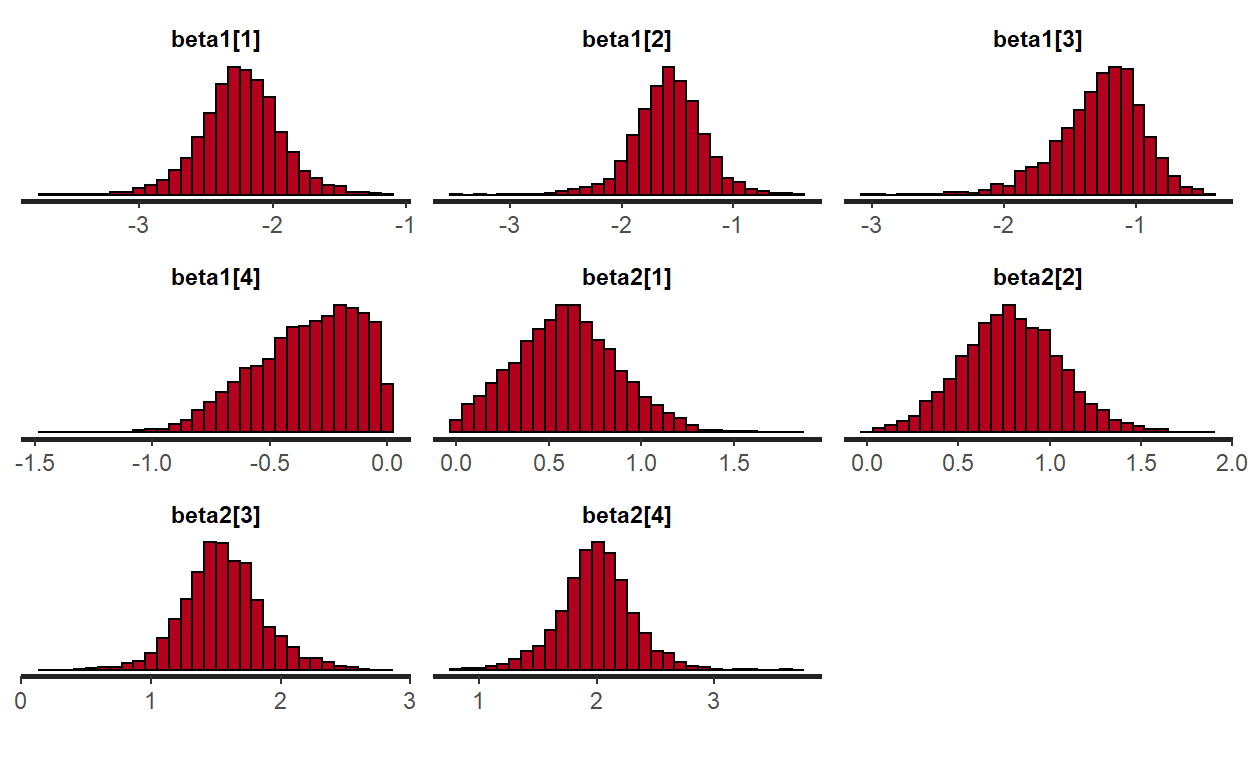plot(fit_hcm2,
plotfun = "hist",
pars = c("gamma","gamma","gamma","gamma",
"gamma","gamma","gamma","gamma"),
inc_warmup = FALSE)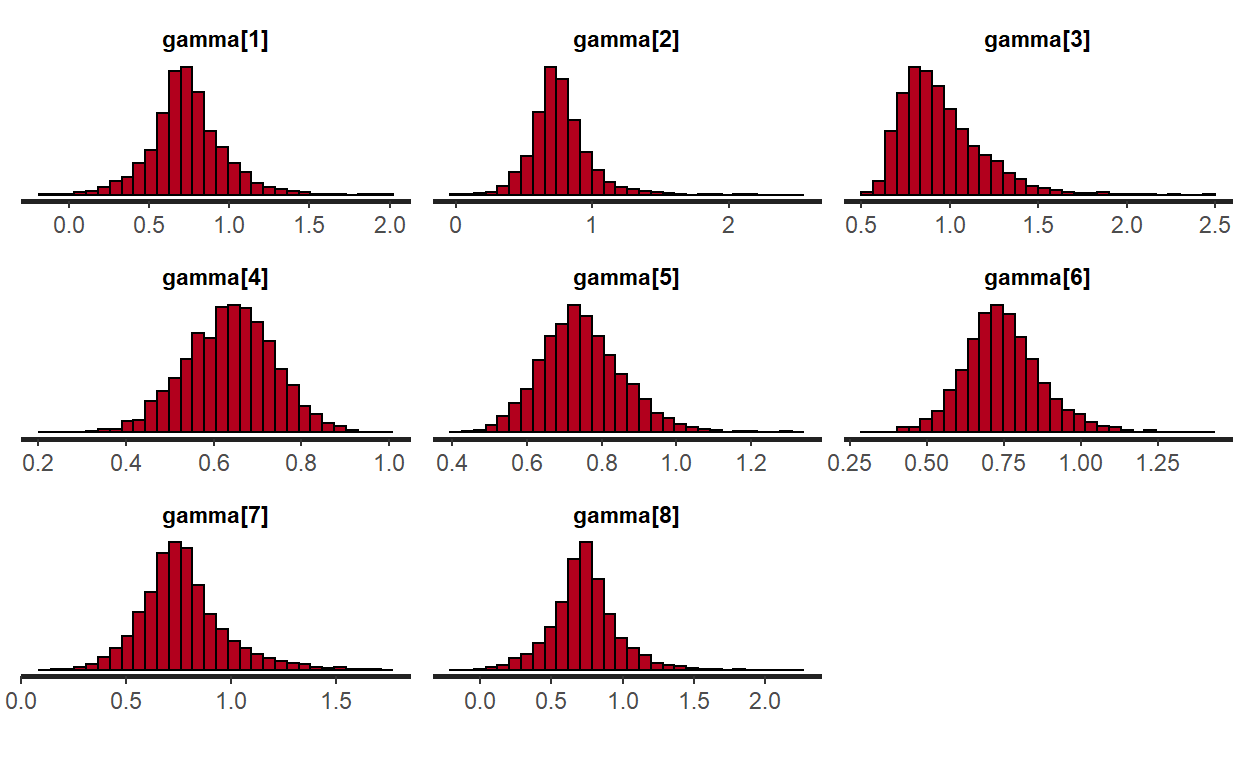Now, this looks very reasonable and much better. Rhat values are within the acceptable range and the posterior distributions look better. If we take the mean of posterior distributions as parameter estimates, they are all within a reasonable range compared to the true values used to generate data.

### Citation

Zopluoglu (2019, Dec. 15). Cengiz Zopluoglu: Fitting Hyperbolic Cosine Model (HCM) For Unfolding Dichotomous Responses using Stan. Retrieved from https://github.com/czopluoglu/website/tree/master/docs/posts/hcm-stan/
@misc{zopluoglu2019fitting,
}